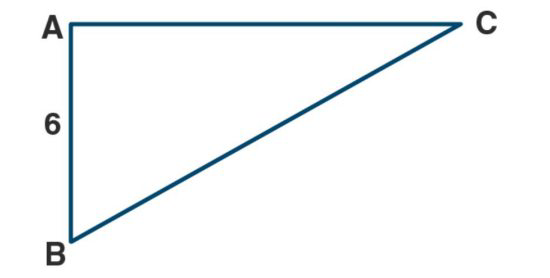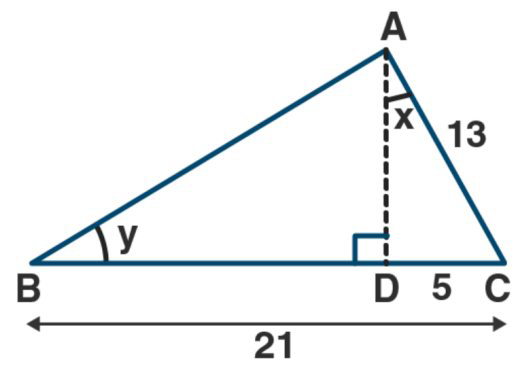Ml aggarwal solutionsOur top 5% students will be awarded a special scholarship to Lido.
Set your child up for success with Lido, book a class today!

(a) From the figure (1) given below, find the values of:

(i) sin B

(i) cos C

(iii) sin B + sin C

(iv) sin B cos C + sin C cos B(b) From the figure (2) given below, find the values of……..

(1) sin B

(ii) cos C• Solution

• Transcript

From the right-angled triangle ABC,

By Pythagoras theorem, we get

\begin{array}{l} \mathrm{BC}^{2}=\mathrm{AC}^{2}+\mathrm{AB}^{2} \\ \mathrm{AC}^{2}=\mathrm{BC}^{2}-\mathrm{AB}^{2} \\ \mathrm{AC}^{2}=(10)^{2}-(6)^{2} \\ \mathrm{AC}^{2}=100-36 \\ \mathrm{AC}^{2}=64 \\ \mathrm{AC}^{2}=8^{2} \\ \mathrm{AC}=8 \end{array}

(i) sin B = perpendicular/ hypotenuse

= AC/BC

= 8/10

= 4/5

(ii) cos C = Base/hypotenuse

= AC/BC

= 8/10

= 4/5

(iii) sin B = Perpendicular/hypotenuse

= AC/BC

= 8/10

= 4/5

Sin C = perpendicular/hypotenuse

= AB/BC

= 6/10

= 3/5

Now,

Sin B + sin C = (4/5) + (3/5)

= (4 + 3)/5

= 7/5

(iv) sin B = 4/5

Cos C = 4/5

Sin C = perpendicular/ hypotenuse

= AB/BC

= 6/10

= 3/5

Cos B = Base/Hypotenuse

= AB/BC

= 6/10

= 3/5

sin B cos C + sin C cos B

= (4/5) × (4/5) × (3/5) × (3/5)

= (26/25) × (9/25)

= (16+9)/25

= 25/25

= 1

From Figure

AC = 13, CD = 5, BC =21,

BD = BC – CD

= 21 – 5

= 16From right angled ∆ACD,

By Pythagoras theorem we get

\begin{array}{l} A C=A D^{2}+C D^{2} \\ A D^{2}=A C^{2}-C D^{2} \end{array}

\begin{array}{l} A D^{2}=(13)^{2}-(5)^{2} \\ A D^{2}=169-25 \\ \left.A D^{2}=144\right) \\ A D^{2}=(12)^{2} \\ A D=12 \end{array}

From right angled ∆ABD,

By Pythagoras angled ∆ABD

By Pythagoras theorem we get

\begin{array}{l} A B^{2}=A D^{2}+B D^{2} \\ A B^{2}=400 \\ A B^{2}=(20)^{2} \\ A B=20 \end{array}

(i) tan x = perpendicular/Base (in right angled ∆ACD)

= 5/12

(ii) cos y = Base/Hypotenuse (in right angled ∆ABD)

= BD/AB

= (20)/12 – (5/3)

Cot y = Base/Perpendicular (in right ∆ABD)

=BD/AB

= 16/20 = 4/5

(iii) ) cos y = Hypotenuse/ perpendicular (in right angled ∆ABD)

BD/AB

= 20/12

= 5/3

Cot y = Base/Perpendicular (in right ∆ABD)

= 16/12

= 4/3

\begin{array}{l} \operatorname{cosec}^{2} y-\cot ^{2} y=(5 / 3)^{2}-(4 / 3)^{2} \\ =(25 / 9)-(16 / 9) \\ =(25-16) / 9 \\ =9 / 9 \\ =1 \end{array}

\text { Hence, } \operatorname{cosec}^{2} y-\cot ^{2} y=1

(iv) sin x = Perpendicular/Hypotenuse (in right angled ∆ACD)

= 12/20

= 3/5

Cot y = Base/Perpendicular (in right angled ∆ABD)

= 16/12

= 4/3

(5/sin x) + (3/sin y) – 3cot y

= 5/(5/13) + 3/(3/5) – 3 × 4/3

= 5 × 13/5 + 3 × 5/3 – 3 × 4/3

= 1 × 13/1 + 1 × 5/1 – 1 × 4/1

= 13 + 5 – 4 = 18 – 4

= 14

Hence , 5/sin x + 3/sin y – 3cot y = 14

Our top 5% students will be awarded a special scholarship to Lido.Lido

Courses

Teachers

Book a Demo with us

Syllabus

Science
English
Coding

Terms & Policies

NCERT Question Bank

Maths
Science

Selina Question Bank

Maths
Physics
Biology

Allied Question Bank

Chemistry